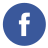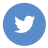### write javascript simple Syntax highlighting code

javascript

Here is an example of a simple JavaScript function that can be used to apply syntax highlighting to a code block:

``````function highlightCode(codeBlock) {
// Split the code block into an array of lines
let lines = codeBlock.split("\n");

// Loop through each line and apply syntax highlighting
for (let i = 0; i < lines.length; i++) {
let line = lines[i];

line = line.replace(/\b(var|let|const)\b/g, '<span class="keyword">\$1</span>');

line = line.replace(/"(.*?)"/g, '<span class="string">"\$1"</span>');

line = line.replace(/\/\/(.*)/g, '<span class="comment">//\$1</span>');

// Add the highlighted line back to the code block
lines[i] = line;
}

// Join the array of lines back into a single string
let highlightedCode = lines.join("\n");

return highlightedCode;
}

// Example usage:
let codeBlock = `
let x = 10;  // Declare a variable
let y = "hello";  // Declare a string
`;

let highlightedCode = highlightCode(codeBlock);
console.log(highlightedCode);``````

This function takes a string containing a code block as an argument and returns a new string with syntax highlighting applied. It does this by using the replace function and regular expressions to search for specific patterns in the code, and then wrapping the matching text in elements with CSS classes applied to add color.

For example, the keyword var is highlighted by searching for the pattern \b(var|let|const)\b and replacing it with \$1. The \$1 in the replacement string refers to the text that was matched by the parentheses in the search pattern.